## A.  Properties and Identities of First and Second Rank Tensors

Several operations and identities of first and second order tensors are defined below for fast consulting. The same notation of the main text is used. The capitalized letters (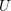,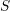,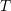) refer to tensors and the amount of bars above them identifies the tensor ranking. The bold lower case letters (,) refer to vectors, while the not bold letters are real numbers, except for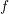which refer to a real function.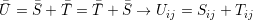(A.1)

##### Product of a Tensor and a Vector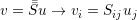(A.2)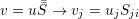(A.3)

##### Product of two Tensors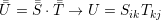(A.4)

##### Transpose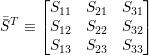(A.5)

##### Trace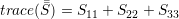(A.6)

##### Inner Product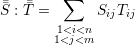(A.7)

##### Tensor Product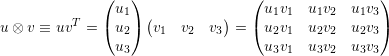(A.8)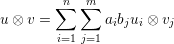(A.9)

In A.9 the vectors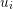and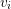are basis of the space ofandrespectively.

##### Tensor Identities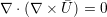(A.10)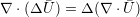(A.11)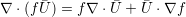(A.12)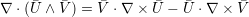(A.13)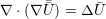(A.14)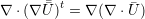(A.15)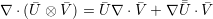(A.16)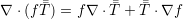(A.17)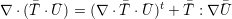(A.18)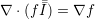(A.19)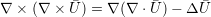(A.20)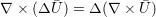(A.21)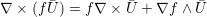(A.22)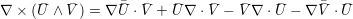(A.23)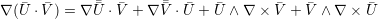(A.24)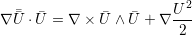(A.25)